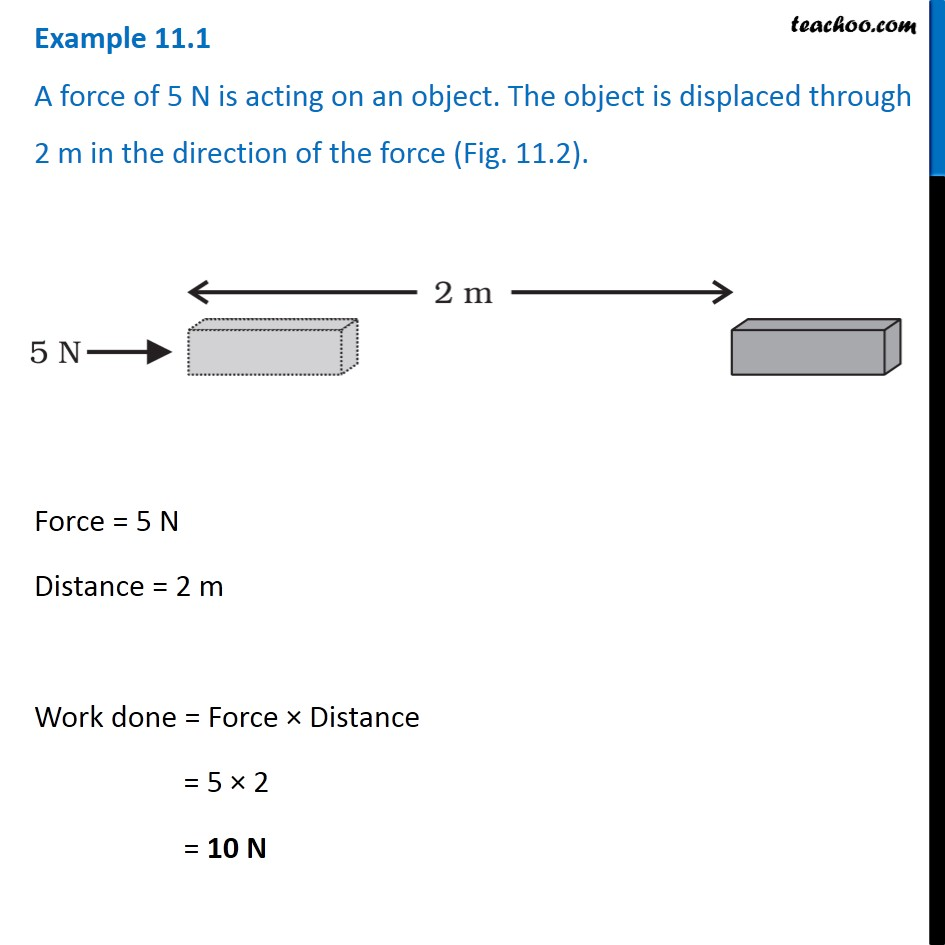Examples from NCERT Book

Class 9
Chapter 11 Class 9 - Work and EnergyLearn in your speed, with individual attention - Teachoo Maths 1-on-1 Class

### Transcript

Example 11.1 A force of 5 N is acting on an object. The object is displaced through 2 m in the direction of the force (Fig. 11.2). Force = 5 N Distance = 2 m Work done = Force × Distance = 5 × 2 = 10 N